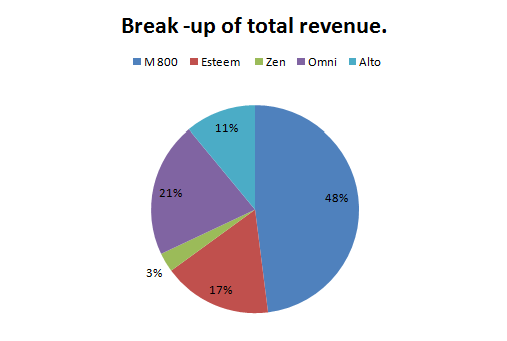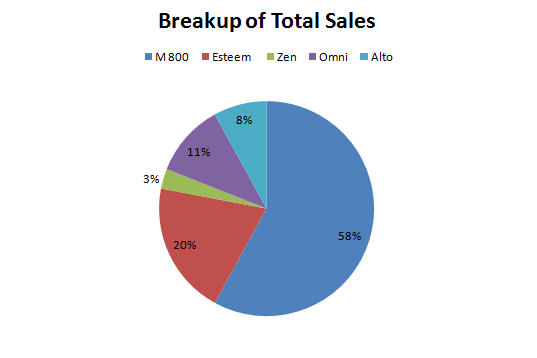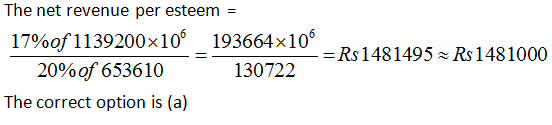Directions: The pie charts below show the break-up of the total revenue and total sales volume for a company for the year ended 2003.
Keep in mind:
Total revenue= Rs. 11,39,200 million
Total sales volume= 653610 unit.
Based on the data provided in both the figures, answer the question that follow.Question 1: What is the net revenue per Esteem sold, approximately?
(a) Rs. 1481000
(b) Rs. 1348000
(c) Rs. 1532000
(d) Rs. 1326000

Question 2: The highest net revenue per vehicle comes from
(a) M 800
(b) Omni
(c) Esteem
(d) Zen

Question 3: If volume of sales of Omni was increased by 25% and this sales increase ‘was’ entirely at the cost of M 800, then what is the percentage decrease in M 800 volume sales?
(a) 1.3%
(b) 2.4%
(c) 4.3%
(d) 4.74%

Question 4: If the net revenue from Alto was to be excluded, what percent of the revenue would Zen form?
(a) 3.27%
(b) 3.17%
(c) 3.37%
(d) 3.47%

Question 5: Which of the following is true?
(i) The sum of the revenue generated by Zen and Omni is lesser than twice that of Alto.
(ii) If the revenue of Esteem and Alto be interchanged, Alto’s revenue per unit would be more than that for Zen.
(iii) The revenue per unit of Esteem is greater than that for M 800.

(a) (i) only
(b) (ii) only
(c) (iii) only
(d) Both (ii) and (iii)The ratio of percentage of revenue to volume

For M 800 = 48/58 = 0.8275

For Omni = 21/11 = 1.909

For Esteem =17/20 = 0.85

For Zen =3/5 = 1

The ratio for Omni is maximum. Hence highest net revenue per vehicle comes from Omni.

Thus, the correct option is (b)

The sale of Omni = 11% of 653610 = 71897

The sale of M 800 = 58% of 653610 = 379094

The new sale of Omni = 71897 × 1.25 = 89871

The increase in the sale of Omni = 89871 – 71897 = 17974 which is the decrease in the sale of M 800

Hence, the required percentage decreases = (17974/379094) x 100 = 4.74%

Alternate Solution (easier to understand and follow):

Let the total volume be 100.

Volume of Omni = 11 and volume of M 800 = 58.

Now the new volume of Omni is 11× 1.25 = 13.75.

Thus, increase = 2.75.

Thus, the decrease of M 800 = 2.75/58 x 100 = 4.74%

The correct option is (d).

Let the total revenue be Rs. 100.

The revenue from Alto = Rs 11 and revenue from Zen = Rs 3

Now if the net revenue from Alto is excluded then the net revenue = 100 – 11 = Rs 89

The required percentage = {(3/89) x 100} = 3.37%

The correct option is (c)

From the pie chart 21+3 > 2 × 11 , thus the sum of Zen and Omni is more than twice of Alto.

Therefore, statement 1 is incorrect.

Now in the second statement, if the revenue of Esteem and Alto be interchanged,

then revenue per unit of Alto = 17/8 = 2.125 and that of Zen is 3/3= = 1.

So, the revenue of Alto is more than that of Zen. Hence, statement 2 is true.

In statement 3, the revenue per unit of Esteem = 17/20 = 0.85 and that of M 800 is 48/58 = 0.83.

Hence, statement 3 is also true.

Thus, option (d) is correct.

### Want to explore more Data Interpretation Sets?

Get Posts Like This Sent to your Email
Updates for Free Live sessions and offers are sent on mail. Don't worry: we do not send too many emails..:)
Get Posts Like This Sent to your Email
Updates for Free Live sessions and offers are sent on mail. Don't worry: we do not send too many emails..:)